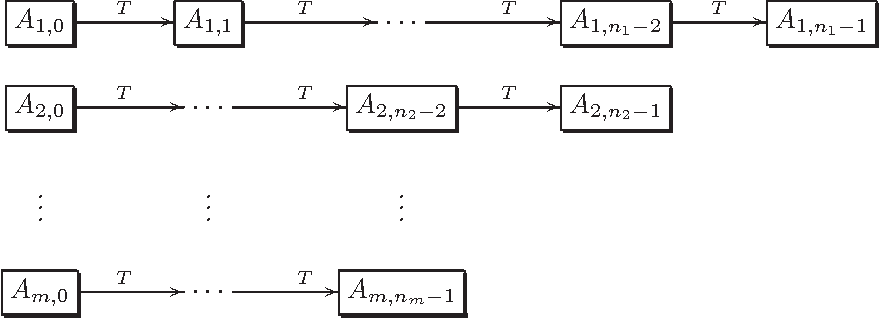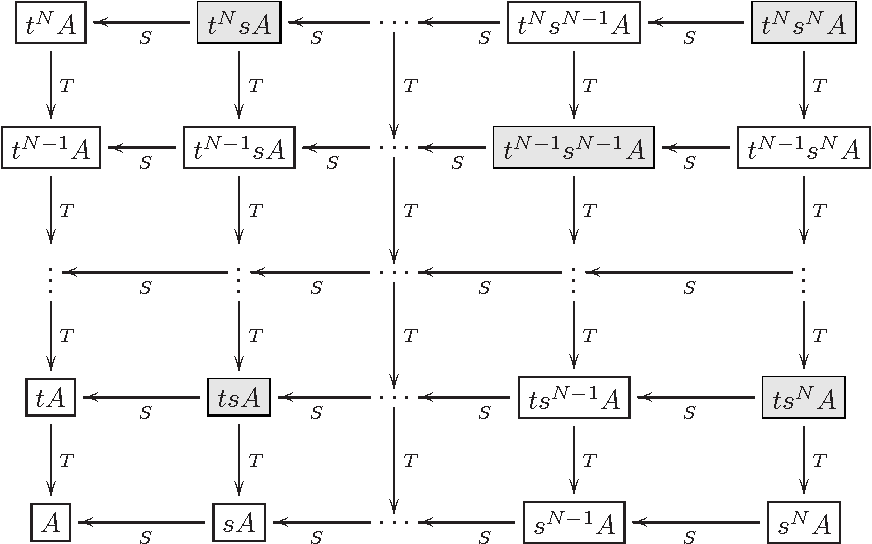You are currently offline. Some features of the site may not work correctly.

# Maximal ergodic theorem

The maximal ergodic theorem is a theorem in ergodic theory, a discipline within mathematics. Suppose that is a probability space, that is a (possibly… Expand
Wikipedia

## Papers overview

Semantic Scholar uses AI to extract papers important to this topic.
2016
2016
• 2016
• Corpus ID: 13748589
In our previous paper (Tirpáková and Markechová in Adv. Differ. Equ. 2015:171, 2015), we presented fuzzy analogies of Mesiar’s… Expand
2014
2014
• 2014
• Corpus ID: 119651793
In , employing the technique of noncommutative interpolation, a maximal ergodic theorem in noncommutative Lp-spaces, 1 < p… Expand
2009
2009
Abstract In this paper, we give a new proof of a result of R. Jones showing almost everywhere convergence of spherical means of… Expand
2009
2009
We provide an elementary proof of Y. Peres' lemma on the existence in certain dynamical systems of what we term heavy points… Expand
••2006
2006
We give a short proof of a strengthening of the Maximal Ergodic Theorem which also immediately yields the Pointwise Ergodic… Expand
1999
1999
In this paper we prove maximal ergodic theorem and a pointwise convergence theorem. Our result is to prove the convergence of Bn… Expand
Highly Cited
1988
Highly Cited
1988
It is shown that the set of squares {n2|n=1, 2,…} or, more generally, sets {nt|n=1, 2,…},t a positive integer, satisfies the… Expand
1983
1983
A new proof of the maximal ergodic theorem is presented. The same idea used in this proof is then used to show that the Hardy… Expand
1966
1966
Introduction. The purpose of this paper is to extend various known results in operator ergodic theory to give a direct approach… Expand
1963
1963
Abstract : The powerful and ingenious method of Chacon and Ornstein (Illinois J. Math. 4:153-160 (1960)), later simplified by E… Expand